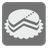Oil Pie
Elementary+
English RUSophia had cooked a pie made from oil for her drones and left it in the kitchen. The Drones were returning home in small groups or by themselves and did not see each other upon their return, so each group did not know how many drones had eaten their slice of the pie. Some groups know about how big the pie was after Sophia removed it from the oven, but other groups don't know this and think the remaining part is the entire pie, then take their portion from the remaining. So each group had divided (mentally) the remaining or initial pie at all and took their part. How many parts will remain after all of the drones return?

Let’s take a look at an example of how this should work: There are 6 drones. The first group consists of 2 drones. They had divided the pie into 6 parts and took 2/6 of the pie. The remainder of the pie is 2/3 of the entire pie. Next returns a single drone, it doesn’t know about the original size of the pie, so it divides the remaining pie into 6 slices and takes 1 part. This leaves 10/18=5/9. The last group is 3 drones, which heard about the original size of the pie from Sophia. They took half of the original pie, so the remaining is 5/9 - 3/6 = 1/18

You are given an ordered array with sizes of the groups in the order they arrived. If a group knows about the initial pie size, then the size is positive. If not, then size will be negative. The recent example will given as (2, -1, 3).divide_pie((2, -1, 3)) == (1, 18) divide_pie((1, 2, 3)) == (0, 1)

How it is used: This mission will familiarize you with operations involving fractions because we must deal with fractions every day of our lives.

Precondition:
all(x for x in groups)
all(-100 < x < 100 for x in groups)